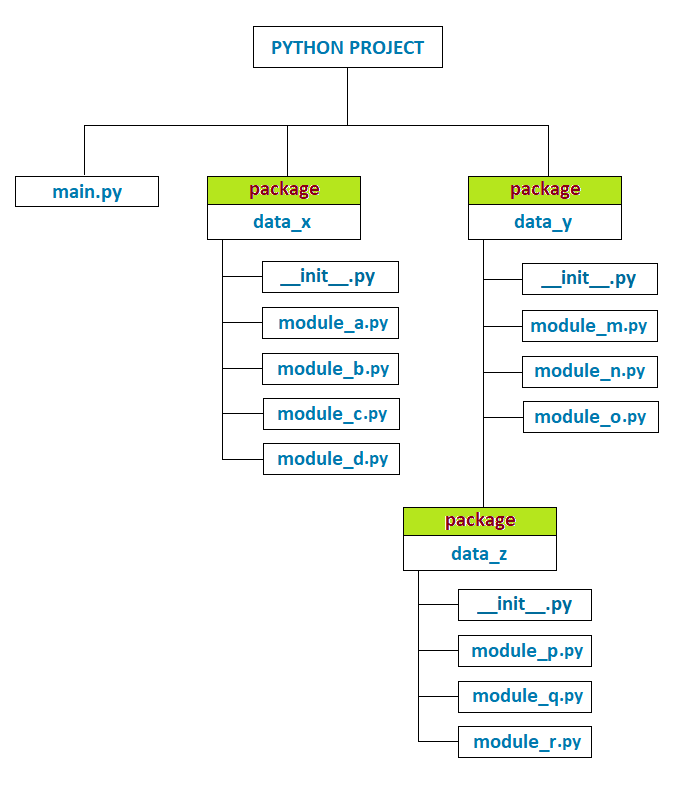# Modules in Python Programming

###### Modules in Python

In this lesson, we will understand what is Module in Python Programming and how to create and implement them along with some examples.

## What is Module in Python?

A Module is simply a regular python file that may contain executable statements, user-defined functions, classes, variables, or other imported modules.

We create modules to store reusable functions and classes so that they can be used in another python program when required just by importing those modules created earlier.## How to Create a Module

To create a module, we have to create a python file and save it with a file extension .py. After that, we will add our reusable functions and classes to this newly created python file. Now this newly created python file containing our function and classes becomes a module that we can import into other python programs.

Let's create a module containing two functions, square and cube, to find the square and cube of a given number and a class that can store an employee's details and saves the file with the name mymodule.py.

### mymodule.py

``````class Employee:
def __init__(self):
self.id = None
self.name = None
self.salary = None

def inputdata(self):
self.id = int(input('Enter ID: '))
self.name = input('Enter Name: ')
self.salary = int(input('Enter Salary: '))

def displaydata(self):
print('Employee ID: %d' %(self.id))
print('Employee Name: %s' %(self.name))
print('Employee Salary: %d' %(self.salary))

def square(n):
return n*n

def cube(n):
return n*n*n``````

## How to Import a Module in Python Program

We can import module in a python program in three different ways and they are:

• import module
• from module import member1, member2...
• from module import *

Let's discuss each of them one by one with examples.

### import module

In this import type, first, we import the module in our program. After that, we call its members (classes and functions), using the module name followed by a dot (.) operator and the member name we want to use in our program.

Let's see an example of how we can import and implement the above-created module mymodule.py in a python program.

### Example of import module

``````import mymodule

x = mymodule.Employee()
x.inputdata()
x.displaydata()

print('Square of 5 = %d' %(mymodule.square(5)))
print('Cube of 2 = %d' %(mymodule.cube(2)))``````

#### Output

```Enter ID: 101
Enter Name: Peter
Enter Salary: 32000
Employee ID: 101
Employee Name: Peter
Employee Salary: 32000
Square of 5 = 25
Cube of 2 = 8```

### from module import member1, member2...

In this import type, we directly import the necessary members from the module in our program. As a result, we can now call the imported members in our program without using their module names.

### Example of from module import member1, member2...

``````from mymodule import cube, square

print('Square of 5 = %d' %(square(5)))
print('Cube of 2 = %d' %(cube(2)))``````

#### Output

```Square of 5 = 25
Cube of 2 = 8```

### from module import *

In this import type, we directly import all the members from the module in our program. As a result, we can now call the imported members in our program without using their module names.

### Example of import module

``````from mymodule import *

x = Employee()
x.inputdata()
x.displaydata()

print('Square of 5 = %d' %(square(5)))
print('Cube of 2 = %d' %(cube(2)))``````

#### Output

```Enter ID: 101
Enter Name: Peter
Enter Salary: 32000
Employee ID: 101
Employee Name: Peter
Employee Salary: 32000
Square of 5 = 25
Cube of 2 = 8```

## Define Alias for Imported Module

Alias is the short name we can define for a module when importing it into our program. It helps us to call the module by its short name instead of its original name.

### Syntax of Module Alias

``import module as alias_name``

### Example of Module Alias

``````import mymodule as mm

x = mm.Employee()
x.inputdata()
x.displaydata()

print('Square of 5 = %d' %(mm.square(5)))
print('Cube of 2 = %d' %(mm.cube(2)))``````

#### Output

```Enter ID: 101
Enter Name: Peter
Enter Salary: 32000
Employee ID: 101
Employee Name: Peter
Employee Salary: 32000
Square of 5 = 25
Cube of 2 = 8```

## Import Module from Package

When we store important modules in a directory, it becomes a package. But to be considered a package in python, the directory must include a file with the name __init__.py, which can be left empty.

Let's assume that we have a python project directory that contains our program file and other packages, as given below in the diagram.We use the dot (.) operator to import a module from a package. For example, to import module_a in main.py from package data_x, we will write the code as:

``from data_x.module_a import *``

To import module_p in main.py from the sub-package data_z, we will write the code as:

``from data_y.data_z.module_p import *``

Note: In the above example, in place of *, we can write the name of the specific members we want to import into our program.

## dir() Function

The dir() function returns the list of an object's valid properties and methods along with built-in properties, which are common for all objects. Here an object can be a list, class, module, etc., and everything is considered an object in python.

### Example

``````import mymodule

print(dir(mymodule))``````

#### Output

```['Employee', '__builtins__', '__cached__', '__doc__', '__file__', '__loader__', '__name__', '__package__', '__spec__', 'cube', 'square']
```

In the above output, names with underscores are the built-in properties, whereas Employee, cube, and square are the members of the module mymodule.